Home > CCA2 > Chapter 12 > Lesson 12.1.3 > Problem12-44

12-44.
1. State the domain and range for each graph below. Then state whether or not it is a function. Homework Help ✎

 a.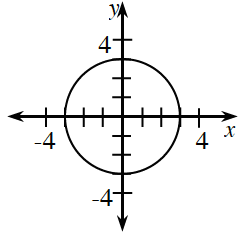b.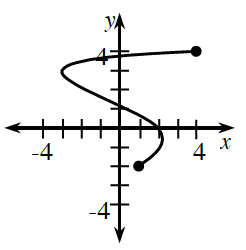c.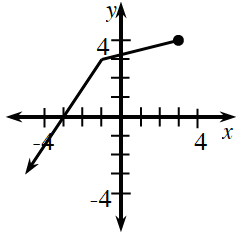d.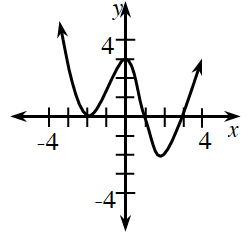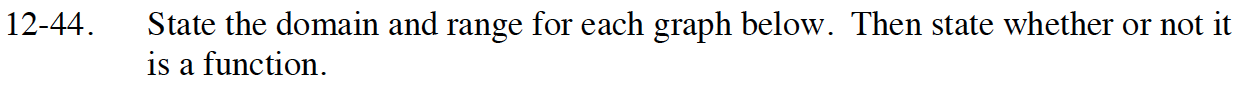The domain is the set of all x-values of a relation or function.
The range is the set of all y-values of a relation or function.
For a relation to be a function, each x-value must correspond to only one y-value.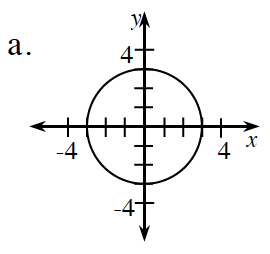Domain: −3 ≤ x ≤ 3
Range: −3 ≤ y ≤ 3
Not a Function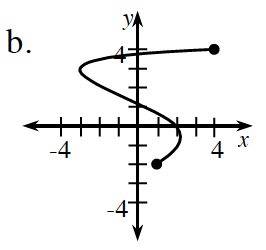Domain: −3 ≤ x ≤ 4
Find the range.
Is this a function?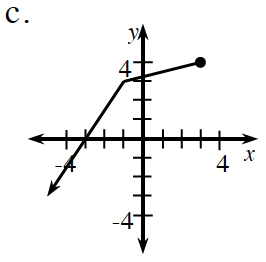Find the domain.
Range: −∞ ≤ y ≤ 4
Is this a function?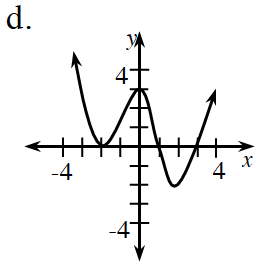This is a function.
Find the domain and range.